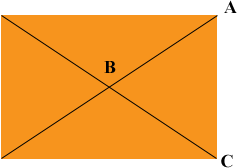SEARCH HOMEMath Central Quandaries & QueriesQuestion from Tabatha, a student: The diagonals of a rectangle are 8 units long and intersect at a 60 degree angle. Find the dimensions of the rectangle.Hi Tabatha,

I can get you started.By the symmetry of the rectangle |AB| = |BC| so triangle ABC is an isosceles triangle. The measure of angle ABC is 60o. What are the measures of angles BCA and CAB? What is the length of CA?

PennyMath Central is supported by the University of Regina and The Pacific Institute for the Mathematical Sciences.